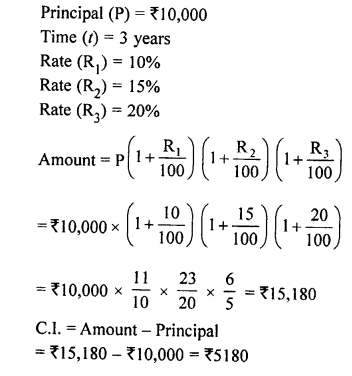## Selina Concise Mathematics Class 8 ICSE Solutions Chapter 9 Simple and Compound Interest

Selina Publishers Concise Mathematics Class 8 ICSE Solutions Chapter 9 Simple and Compound Interest

ICSESolutions.com provides step by step solutions for Selina Concise ICSE Solutions for Class 8 Mathematics Chapter 9 Simple and Compound Interest. You can download the Selina Concise Mathematics ICSE Solutions for Class 8 with Free PDF download option. Selina Publishers Concise Mathematics for Class 8 ICSE Solutions all questions are solved and explained by expert mathematic teachers as per ICSE board guidelines.

### Simple and Compound Interest Exercise 9A – Selina Concise Mathematics Class 8 ICSE Solutions

Question 1.
Find the interest and the amount on:
(i) ₹ 750 in 3 years 4 months at 10% per annum.
(ii) ₹ 5,000 at 8% per year from 23rd December 2011 to 29th July 2012.
(iii) ₹ 2,600 in 2 years 3 months at 1% per month.
(iv) ₹ 4,000 in 1$$\frac { 1 }{ 3 }$$ years at 2 paise per rupee per month.
Solution: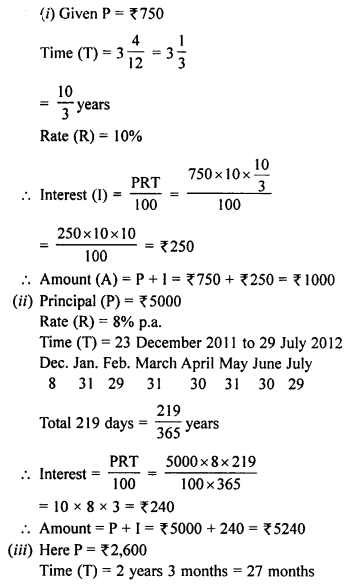Question 2.
Rohit borrowed Rs. 24,000 at 7.5 percent per year. How much money will he pay at the end of 4th years to clear his debt ?
Solution: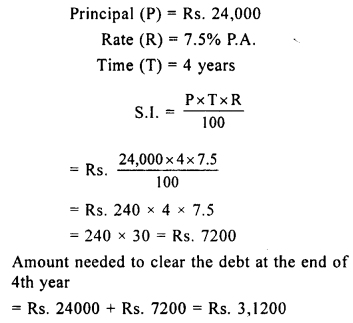Question 3.
The interest on a certain sum of money is Rs. 1,480 in 2 years and at 10 per cent per year. Find the sum of money.
Solution:
Let P = Rs. x
Time (T) = 2 years
Rate (R) = 0%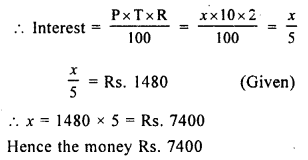Question 4.
On what principal will the simple interest be Rs. 7,008 in 6 years 3 months at 5% per year ?
Solution: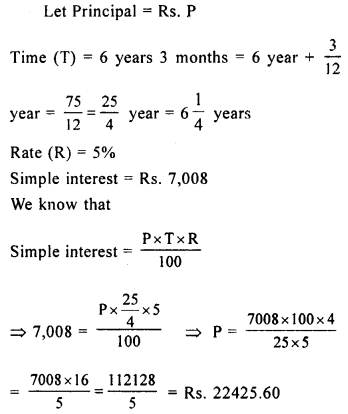Question 5.
Find the principal which will amount to Rs. 4,000 in 4 years at 6.25% Per annum.
Solution: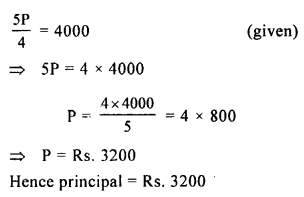Question 6.
(i) At what rate per cent per annum will Rs. 630 produce an interest of Rs. 126 in 4 years ?
(ii) At what rate per cent per year will a sum double itself in 6$$\frac { 1 }{ 4 }$$ years ?
Solution:Question 7.
(i) In how many years will Rs.950 produce Rs.399 as simple interest at 7% ?
(ii) Find the time in which Rs.1200 will amount to Rs.1536 at 3.5% per year.
Solution: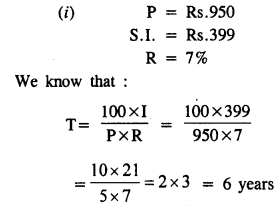Question 8.
The simple interest on a certain sum of money is $$\frac { 3 }{ 8 }$$ of the sum in 6$$\frac { 1 }{ 4 }$$ years. Find the rate percent charged.
Solution: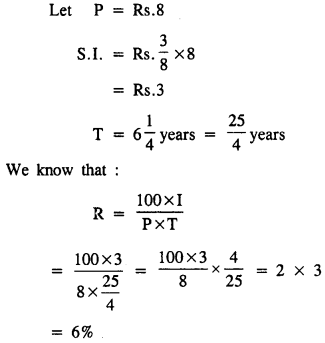Question 9.
What sum of money borrowed on 24th May will amount to Rs. 10210.20 on 17th October of the same year at 5 percent per annum simple interest.
Solution:
A = Rs. 10210.20
R = 5% P.A.
T=May + June + July + August + Sept.+ Oct.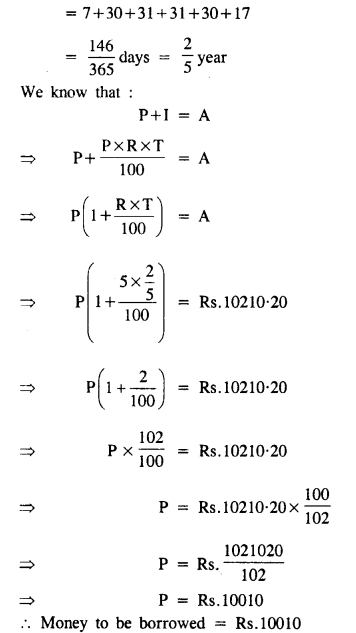Question 10.
In what time will the interest on a certain sum of money at 6% be $$\frac { 5 }{ 8 }$$ of itself ?
Solution: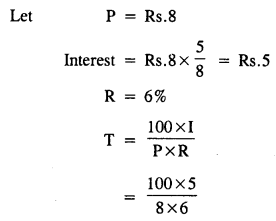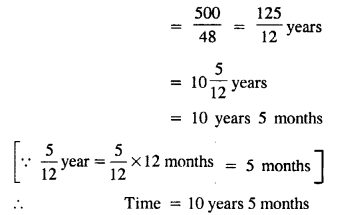Question 11.
Ashok lent out Rs.7000 at 6% and Rs.9500 at 5%. Find his total income from the interest in 3 years.
Solution:Question 12.
Raj borrows Rs.8,000; out of which Rs. 4500 at 5% and remainder at 6%. Find the total interest paid by him in 4 years.
Solution: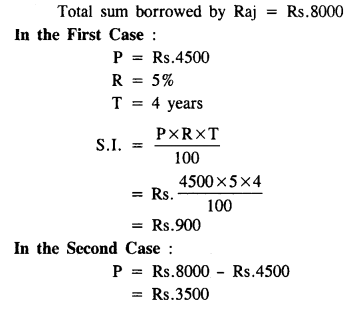Question 13.
Mohan lends Rs.4800 to John for 4$$\frac { 1 }{ 2 }$$ years and Rs.2500 to Shy am for 6 years and receives a total sum of Rs.2196 as interest. Find the rate percent per annum, it being the same in both the cases.
Solution: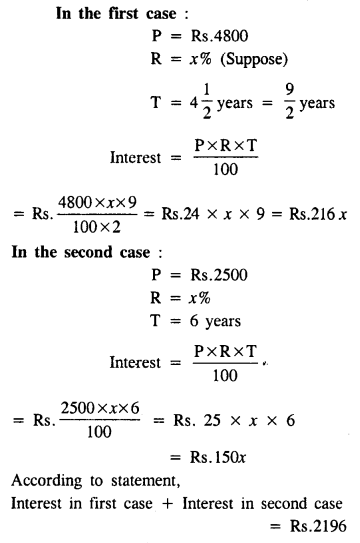Question 14.
John lent Rs. 2550 to Mohan at 7.5 per cent per annum. If Mohan discharges the debt after 8 months by giving an old black and white television and Rs. 1422.50; find the price of the television.
Solution: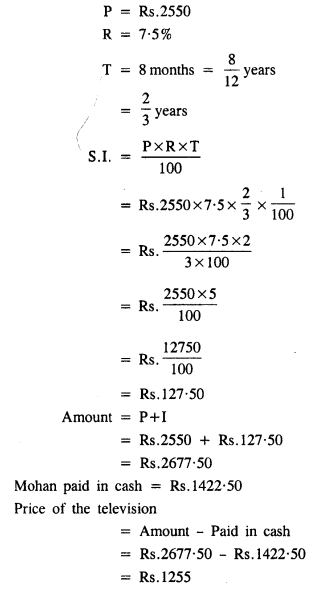### Simple and Compound Interest Exercise 9B – Selina Concise Mathematics Class 8 ICSE Solutions

Question 1.
The interest on a certain sum of money is 0.24 times of itself in 3 years. Find the rate of interest.
Solution:Question 2.
If ₹ 3,750 amount to ₹ 4,620 in 3 years at simple interest. Find:
(i) the rate of interest
(ii) the amount of Rs. 7,500 in 5$$\frac { 1 }{ 2 }$$ years at the same rate of interest
Solution: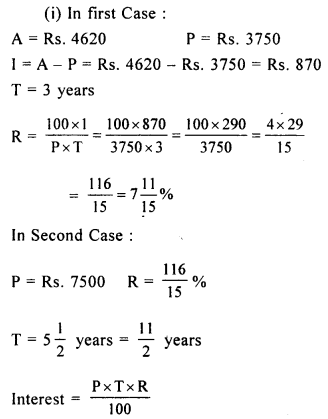Question 3.
A sum of money, lent out at simple interst, doubles itself in 8 years. Find :
(i) the rate of interest
(ii) in how many years will the sum become triple (three times) of itself at the same rate per cent ?
Solution: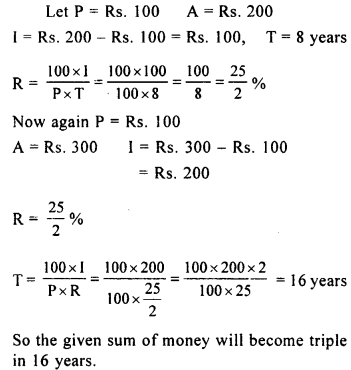Question 4.
Rupees 4000 amount to Rs.5000 in 8 years ; in what time will Rs.2100 amount to Rs.2800 at the same rate ?
Solution: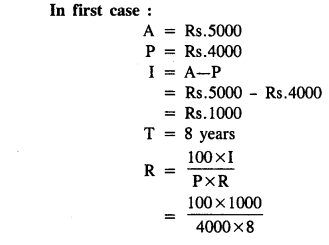Question 5.
What sum of money lent at 6.5% per annum will produce the same interest in 4 years as Rs.7500 produce in 6 years at 5% per annum ?
Solution: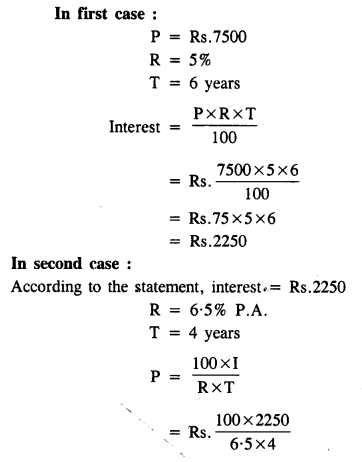Question 6.
A certain sum amounts to Rs.3825 in 4 years and to Rs.4050 in 6 years. Find the rate percent and the sum.
Solution: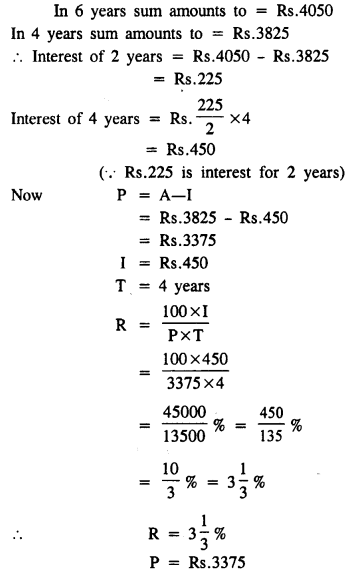Question 7.
At what rafepercent of simple interest will the interest on Rs.3750 be one-fifth of itself in 4 years ? To what will it amount in 15 years ?
Solution: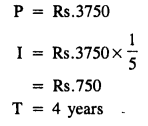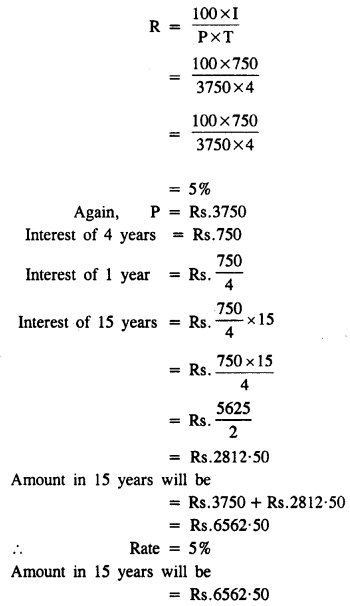Question 8.
On what date will ₹ 1950 lent on 5th January, 2011 amount to ₹ 2125.50 at 5 percent per annum simple interest?
Solution: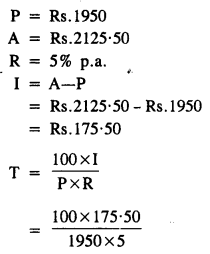Question 9.
If the interest on Rs.2400 be more than the interest on Rs.2000 by Rs.60 in 3 years at the same rate percent ; find the rate.
Solution: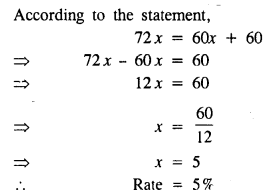Question 10.
Divide Rs. 15,600 into two parts such that the interest on one at 5 percent for 5 years may be equal to that on the other at 4$$\frac { 1 }{ 2 }$$ per cent for 6 years.
Solution:### Simple and Compound Interest Exercise 9C – Selina Concise Mathematics Class 8 ICSE Solutions

Question 1.
A sum of Rs. 8,000 is invested for 2 years at 10% per annum compound interest. Calculate:
(i) interest for the first year.
(ii) principal for the second year.
(iii) interest for the second year.
(iv) final amount at the end of second year
(v) compound interest earned in 2 years.
Solution:
(i) Here Principal (P) = Rs. 8,000
Rate of interest = 10%Question 2.
A man borrowed Rs. 20,000 for 2 years at 8% per year compound interest. Calculate :
(i) the interest of the first year.
(ii) the interest of the second year.
(iii) the final amount at the end of second year.
(iv) the compound interest of two years.
Solution: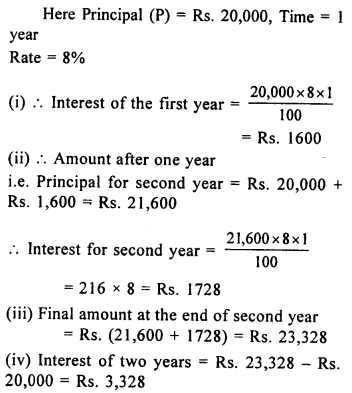Question 3.
Calculate the amount and the compound interest on Rs. 12,000 in 2 years and at 10% per year.
Solution: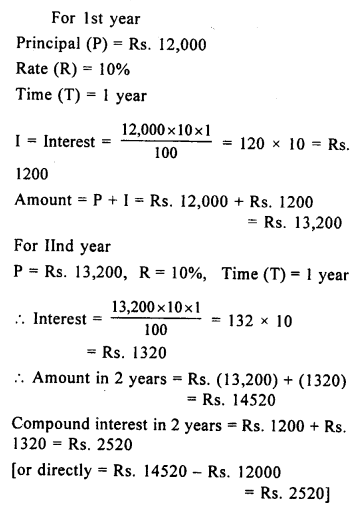Question 4.
Calculate the amount and the compound interest on Rs. 10,000 in 3 years at 8% per annum.
Solution: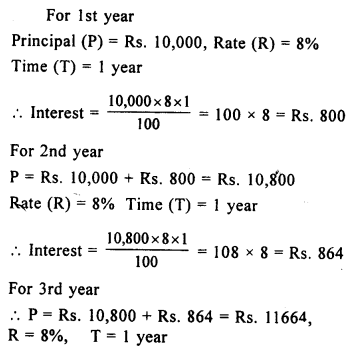Question 5.
Calculate the compound interest on Rs. 5,000 in 2 years ; if the rates of interest for successive years be 10% and 12% respectively.
Solution:Question 6.
Calculate the compound interest on Rs. 15,000 in 3 years ; if the rates of interest for successive years be 6%, 8% and 10% respectively.
Solution: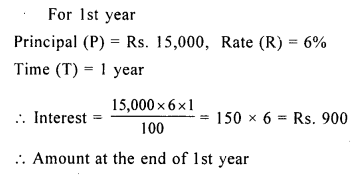Question 7.
Mohan borrowed Rs. 16,000 for 3 years at 5% per annum compound interest. Calculate the amount that Mohan will pay at the end of 3 years.
Solution: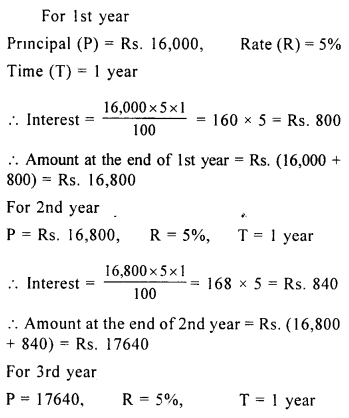Question 8.
Rekha borrowed Rs. 40,000 for 3 years at 10% per annum compound interest. Calculate the interest paid by her for the second year.
Solution:Question 9.
Calculate the compound interest for the second year on Rs. 15000 invested for 5 years at 6% per annum.
Solution:= 159 x 6 = Rs. 954

Question 10.
A man invests Rs. 9600 at 10% per annum compound interest for 3 years. Calculate :
(i) the interest for the first year.
(ii) the amount at the end of the first year.
(iii) the interest for the second year.
(iv) the interest for the third year.
Solution:Question 11.
A person invests Rs. 5,000 for two years at a certain rate of interest compounded annually. At the end of one year, this sum amounts to Rs. 5,600. Calculate :
(i) the rate of interest per year.
(ii) the amount at the end of the second year.
Solution:Question 12.
Calculate the difference between the compound interest and the simple interest on ₹ 7,500 in two years and at 8% per annum.
Solution:Question 13.
Calculate the difference between the compound interest and the simple interest on ₹ 8,000 in three years and at 10% per annum.
Solution:Question 14.
Rohit borrowed ₹ 40,000 for 2 years at 10% per annum C.I. and Manish borrowed the same sum for the same time at 10.5% per annum simple interest. Which of these two gets less interest and by how much?
Solution: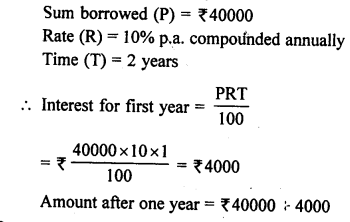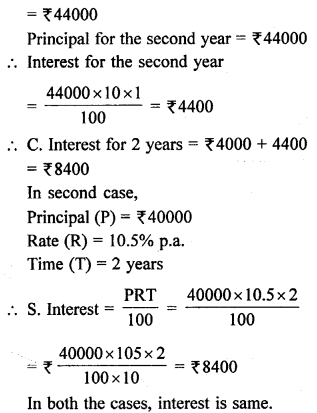Question 15.
Mr. Sharma borrowed ₹ 24,000 at 13% p.a. simple interest and an equal sum at 12% p.a. compound interest. Find the total interest earned by Mr. Sharma in 2 years.
Solution: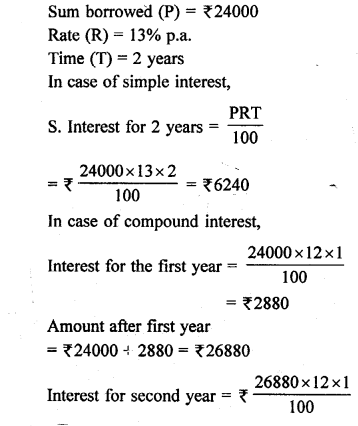Question 16.
Peter borrows ₹ 12,000 for 2 years at 10% p.a. compound interest. He repays ₹ 8,000 at the end of first year. Find:
(i) the amount at the end of first year, before making the repayment.
(ii) the amount at the end of first year, after making the repayment.
(iii) the principal for the second year.
(iv) the amount to be paid at the end of second year, to clear the account.
Solution:Question 17.
Gautam takes a loan of ₹ 16,000 for 2 years at 15% p.a. compound interest. He repays ₹ 9,000 at the end of first year. How mucH must he pay at the end of second year to clear the debt?
Solution:
Loan taken (P) = ₹ 16000
Rate (R) = 15% p.a.
Time (T) = 2 yearsQuestion 18.
A certain sum of money, invested for 5 years at 8% p.a. simple interest, earns an interest of ₹ 12,000. Find:
(i) the sum of money.
(ii) the compound interest earned by this money in two years and at 10% p.a. compound interest.
Solution:Question 19.
Find the amount and the C.I. on ₹ 12,000 at 10% per annum compounded half-yearly.
Solution:Question 20.
Find the amount and the C.I. on ₹ 8,000 in 1$$\frac { 1 }{ 2 }$$ years at 20% per year compounded half- yearly.
Solution: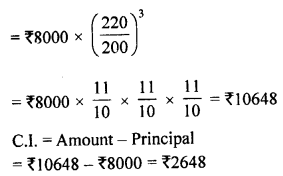Question 21.
Find the amount and the compound interest on ₹ 24,000 for 2 years at 10% per annum compounded yearly.
Solution:Question 22.
Find the amount and the compound interest on ₹ 16,000 for 3 years at 5% per annum compounded annually.
Solution: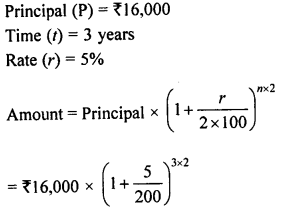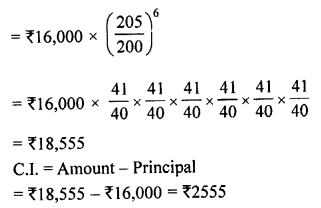Question 23.
Find the amount and the compound interest on ₹ 20,000 for 1$$\frac { 1 }{ 2 }$$ years at 10% per annum compounded half-yearly.
Solution:Question 24.
Find the amount and the compound interest on ₹ 32,000 for 1 year at 20% per annum compounded half-yearly.
Solution: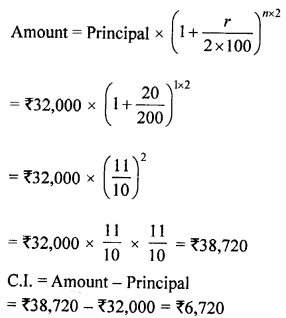Question 25.
Find the amount and the compound interest on ₹ 4,000 in 2 years, if the rate of interest for first year is 10% and for the second year is 15%.
Solution: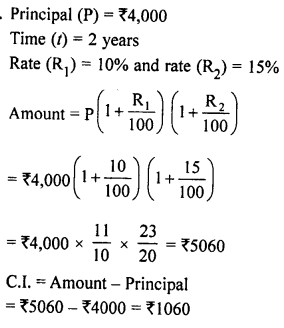Question 26.
Find the amount and the compound interest on ₹ 10,000 in 3 years, if the rates of interest for the successive years are 10%, 15% and 20% respectively.
Solution: